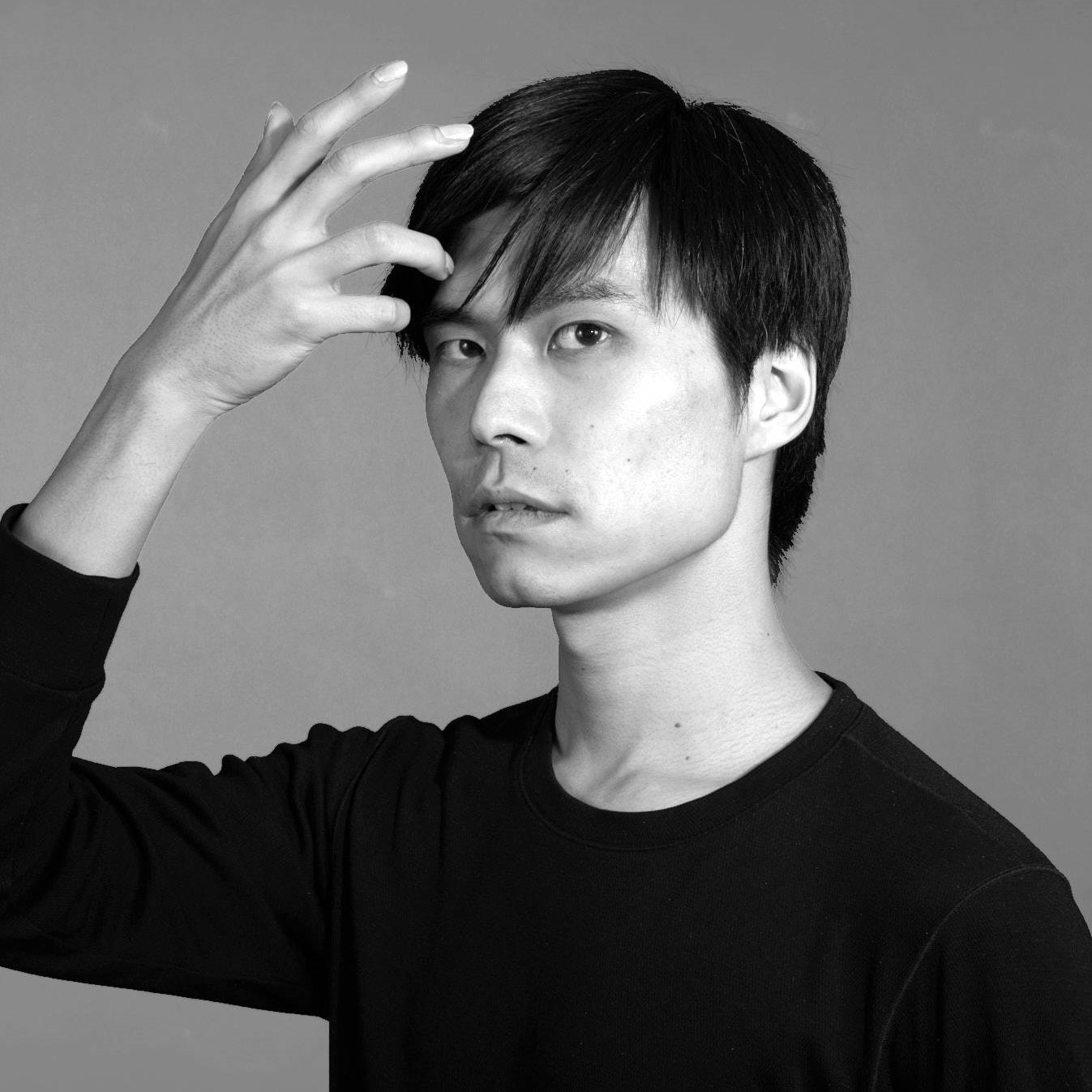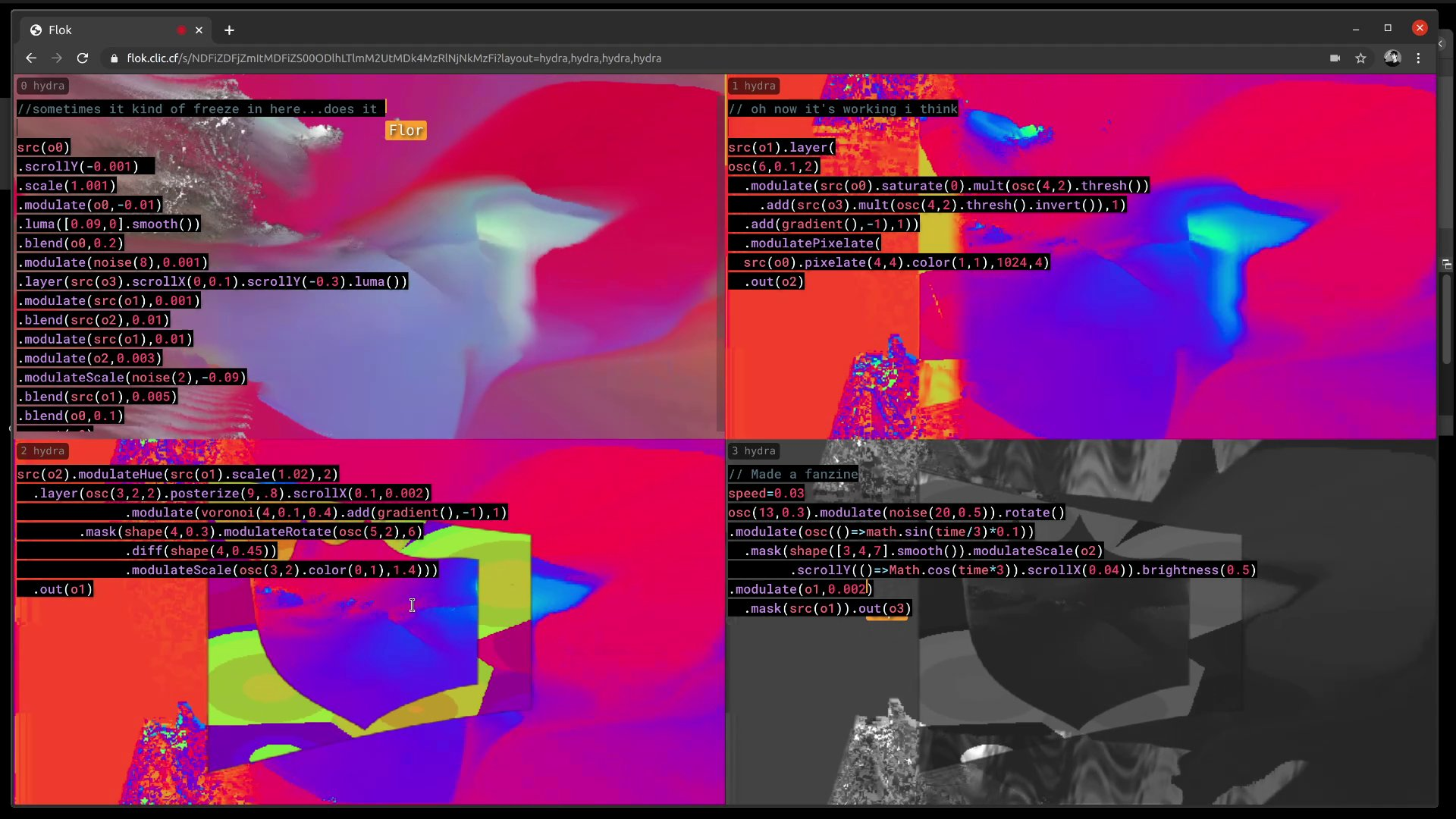Naoto Naoto Hieda

# Thoughts on Hydra Modulate FunctionHydra is an analog-synth-like coding environment for real-time visuals. It is created by Olivia Jack and is open-source.

One of the key function of Hydra is `modulate`. As referenced in Hydra README, Lumen is a commercial software that also uses modulation, and it has a documentation about modulation.

```osc(20,0.1,2) .modulate(noise(), () => time%1*0.1, 0.1) .out()```

I usually think modulation as a way to push pixels in x and y directions based on the red and green channels of the input texture. In the example above, the original texture (rainbow oscillator) is pushed by an input texture (noise). Let’s take a look another example.

`shape(4).modulate(noise(), () => time%1*0.1, 0.1).out()`

Here, the square shape is modulated in both x, y directions; in fact, `noise` returns grayscale values, so pixels are pushed equally in x and y directions. Effectively, pixels are pushed in a diagonal direction. To modulate only in x direction, green channel should be discarded (there is also `modulateScrollX` which specifically modulates in x direction, but currently there is a bug that the function is currently broken):

```shape(4) .modulate(noise().color(1,0), () => time%1*0.1, 0.1) .out()```

Notice that while the effect is similar, the top and bottom edges of the square are preserved because modulation only applies in the x (horizontal) direction. You might wonder why I used `color(1,0)` instead of `color(1,0,0)`. The reason is that modulate only looks at the red and green channels, so the value in the blue channel does not matter. In `modulate` family, the following functions use only 1 channel (red):

• `modulateRepeatX`
• `modulateRepeatY`
• `modulateScrollX`
• `modulateScrollY`
• `modulateKaleid`
• `modulateRotate`

note that all the functions samples red channel; for example, `modulateScrollY` uses red channel to shift pixels in y direction while `modulate` uses red for x and green channel for y direction.

The following functions use 2 channels (red and green):

• `modulate`
• `modulateRepeat`
• `modulateScale`
• `modulatePixelate`

At last, `modulateHue` uses 3 channels (red, green and blue).

Therefore, blue channel will not affect the results of `modulate`. For example, `gradient` takes an argument to “animate” the texture.

`gradient(2).out()`

Using `gradient` animation as an input does not animate the texture because the animation only happens in the blue channel:

```shape(4) .modulate(gradient(2), 0.1) .out()```

How can we use the animation of `gradient`? Although swapping color channels can be confusing in Hydra, you can simply use `hue` to effectively shift values between channels:

```shape(4) .modulate(gradient(2).hue(), 0.1) .out()```

This is where things get confusing. `hue` is supposed to change color but in the code above, what is changed is the direction of pixels to push. Let’s look at an even more confusing example:

```render() osc(30,0,2) .modulateScale(shape(99,0.5),0.5,1) .out(o0) osc(30,0,2) .modulateScale(shape(99,0.5).color(0.5,0.5),1,1) .out(o1) osc(30,0,2) .modulateScale(shape(99,0.5).brightness(2),0.5,0) .out(o2) osc(30,0,2) .modulateScale(shape(99,0.5).invert(),-0.5,1.5) .out(o3) ```

While they all have different operations using `color`, `brightness` and `invert`, with an appropriate arguments of `modulateScale`, they all yield the same texture. This is because that the color operations are simply arithmetic operations (`color` for `*`, `brightness` for `+` and `invert` for `-`).

By understanding such operations, animations can be coded without array objects or arrow functions:

```shape(4) .modulateScale(gradient().r() .scrollX(0,1).pixelate(1,1),1,1) .out() ```

which is identical to

`shape(4).scale(()=>time%1+1).out()`

(`r()` copies the red channel to green and blue channels; thus scaling is applied equally to x and y directions). I prefer the color operations inside modulation because they give more freedom to work in the spatial domain:

``` shape(4) .modulateScale(gradient().r() .scrollX(0,1).pixelate(8,1),1,1) .out() ```

Nevertheless, I am hesitant to tell people about these tricks because simply they are not intuitive. As a design question, I would love to ask people what would be an alias of `color` inside modulation to actually multiply the values for modifying the texture coordinates? `scale` may sound like a good idea, but unfortunately it is already taken by real scaling function that stretches the texture in the spatial domain.

This is not totally a hydra geek talk. In the following example of naive uses of modulation family functions, the pairs look similar to each other:

```render() osc(30,0,0) .modulate(noise(3)) .out(o0) osc(30,0,0) .modulateRotate(noise(3)) .out(o1) osc(30,0,0) .modulatePixelate(noise(3)) .out(o2) osc(30,0,0) .modulateKaleid(noise(3)) .out(o3) ```

Based on the observations above, these modulators become controllable by adding a few color operations and by picking the right parameters:

```render() osc(30,0,0) .modulate(noise(3)) .out(o0) osc(30,0,0) .modulateRotate(noise(3).mult(shape(99,0.0,0.7)),Math.PI*2,0) .out(o1) osc(30,0,0) .modulatePixelate(noise(3).pixelate(8,8),1024,8) .out(o2) osc(30,0,0) .modulateKaleid(noise(3).color(0.1),4) .out(o3) ```

I would love to contribute to Hydra (or specifically hydra-synth) to make modulators more accessible. However, as written above, it is not a simple engineering problem but it needs to be closely discussed by community members to make it accessible.

comments powered by Disqus